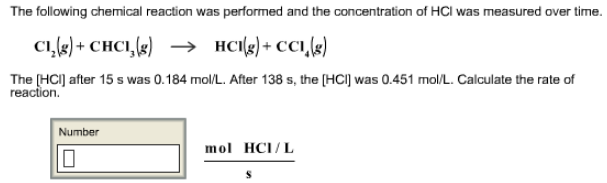# Problem: The following chemical reaction was performed and the concentration of HCl was measured over time. Cl2 (g) + CHCl3 (g) → HCl (g) + CCl4 (g) The [HCI] after 15 s was 0.184 mol/L. After 138 s, the [HCI] was 0.451 mollL. Calculate the rate of reaction.

🤓 Based on our data, we think this question is relevant for Professor Woods' class at SLU.

###### FREE Expert Solution###### Problem Details

The following chemical reaction was performed and the concentration of HCl was measured over time.

Cl2 (g) + CHCl3 (g) → HCl (g) + CCl4 (g)

The [HCI] after 15 s was 0.184 mol/L. After 138 s, the [HCI] was 0.451 mollL. Calculate the rate of reaction.